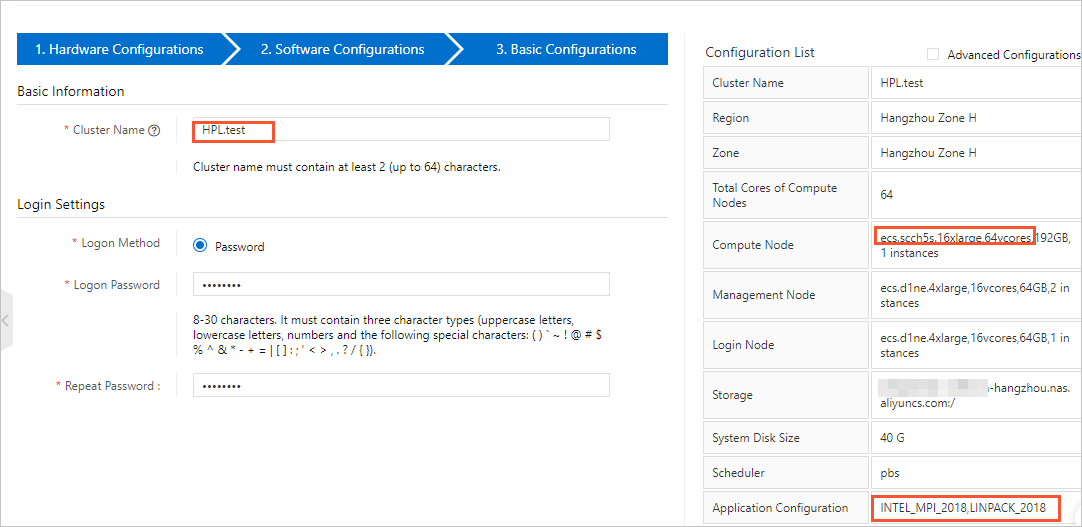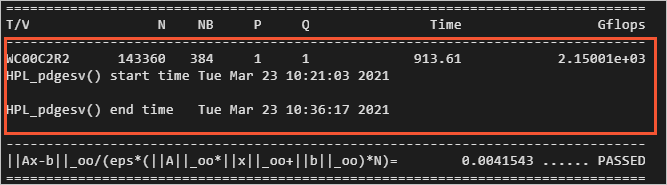Search
Document Center

# Use the HPL benchmark to test the FLOPS of an E-HPC cluster

Last Updated: May 19, 2022

This topic describes how to use the High-Performance Linpack (HPL) benchmark to test the floating-point operations per second (FLOPS) of an E-HPC cluster.

## Background information

HPL is a benchmark that is used to test the FLOPS of high-performance computing (HPC) clusters. HPL evaluates the floating-point computing power of HPC clusters. The evaluation is based on a test for solving dense linear unary equations of Nth degree by using Gaussian elimination.

The peak FLOPS is the number of floating-point operations that a computer can perform per second. The peak FLOPS can be divided into two types, theoretical peak FLOPS and actual peak FLOPS. The theoretical peak FLOPS is the number of floating-point operations that a computer can theoretically perform per second. The theoretical peak FLOPS is determined by the clock speed of the CPU. The theoretical peak FLOPS is calculated by using the following formula: Theoretical peak FLOPS = Clock speed of the CPU × Number of CPU cores × Number of floating-point operations that the CPU performs per cycle. This topic describes how to test the actual peak FLOPS by using HPL.

## Before you begin

Before the test, prepare an example file named HPL.dat on your computer. The file includes the runtime parameters of HPL. The following example shows the recommended configurations that are used to run HPL on a scch5s instance.

``````HPLinpack benchmark input file
Innovative Computing Laboratory, University of Tennessee
HPL.out      output file name (if any)
6            device out (6=stdout,7=stderr,file)
1            # of problems sizes (N)
143360 256000 1000         Ns
1            # of NBs
384 192 256      NBs
1            PMAP process mapping (0=Row-,1=Column-major)
1            # of process grids (P x Q)
1 2          Ps
1 2          Qs
16.0         threshold
1            # of panel fact
2 1 0        PFACTs (0=left, 1=Crout, 2=Right)
1            # of recursive stopping criterium
2            NBMINs (>= 1)
1            # of panels in recursion
2            NDIVs
1            # of recursive panel fact.
1 0 2        RFACTs (0=left, 1=Crout, 2=Right)
0            BCASTs (0=1rg,1=1rM,2=2rg,3=2rM,4=Lng,5=LnM)
0            DEPTHs (>=0)
0            SWAP (0=bin-exch,1=long,2=mix)
1            swapping threshold
1            L1 in (0=transposed,1=no-transposed) form
1            U  in (0=transposed,1=no-transposed) form
0            Equilibration (0=no,1=yes)
8            memory alignment in double (> 0)``````

During the test, you can reset the parameters in the HPL.dat file based on the hardware settings of the node. The following examples describe the parameters.

• Content in line 5 and line 6:

``````1            # of problems sizes (N), where N indicates the size of matrices that you want to solve.
143360 256000 1000         Ns  ``````

N indicates the size of matrices that you want to solve. A larger matrix size N indicates a greater proportion of valid operations to all operations. Therefore, a larger N reflects a higher FLOPS of the system. However, a larger matrix size leads to higher memory usage. If the available memory space of the system becomes insufficient, the cache is used instead. Therefore, the system performance is greatly reduced. The optimal usage of system memory that the matrix occupies is about 80%. The following formula is used to calculate the value of N: N × N × 8 = Total system memory × 80%. The unit of the total memory is bytes.

• Content in line 7 and line 8:

``````1            # of NBs, where NB indicates the size of block matrices when the matrices are solved.
384 192 256      NBs ``````

The size of block matrices when the matrices are solved. The block size has a major impact on the system performance. The value of NB is affected by multiple factors, such as hardware and software. The optimal value of NB is obtained from actual tests. The value of NB meets the following conditions:

• The value of NB can neither be too large nor too small. In most cases, the value is less than 384.

• The product of NB × 8 must be a multiple of the number of cache lines.

• The value of NB is determined by multiple factors, such as the communication mode, matrix size, network conditions, and clock speed.

You can obtain several appropriate NB values from single-node or single-CPU tests. However, if the system capacity is increased and a larger memory space is required, some of these NB values may lead to a decrease in FLOPS. Therefore, we recommend that you select three NB values that can lead to satisfactory FLOPS in small-scale tests. This way, you can perform large-scale tests to decide the optimal NB value.

• Content in line 10, line 11 and line 12:

``````1            # of process grids (P x Q), where P indicates the number of processors for rows, and Q indicates the number of processors for columns.
1 2          Ps
1 2          Qs  ``````

P indicates the number of processors for rows, and Q indicates the number of processors for columns. The product of P and Q represents a two-dimensional processor grid. Formula: P × Q= Number of CPUs = Number of processes In most cases, the FLOPS is optimal if one CPU handles one process. For Intel ®Xeon ®, you can improve HPL performance by disabling hyper-Threading. In most cases, the values of P and Q meet the following conditions:

• P ≤ Q. In most cases, the value of P is less than the value of Q. This is because the number and data volume of communications in columns are much greater than those in rows.

• We recommend that you set the value of P to an exponential power of 2. In HPL, binary exchange is used for horizontal communication. The FLOPS is optimal when the number of processors (P) in the horizontal direction is equal to a power of 2.

## Procedure

1. Log on to the E-HPC console.

2. Create a cluster named HPL.test.

• Compute Node: Select an SCC instance type, for example, ecs.scch5s.16xlarge.

• Other Software: Install linpack 2018 and intel-mpi 2018.

Note

You can also install the preceding software in an existing cluster. For more information, see Install software.3. Create a sudo user named hpltest.

4. Create a job file.

1. In the left-side navigation pane, click Job.

2. Select a cluster from the Cluster drop-down list. Then, click Create Job.

3. On the Create Job page, choose Create File > Open Local File.

4. In the local directory of your computer, find the HPL.dat file, and click Open.

5. Create a job script and submit the job.

1. On the Create Job page, choose Create File > Template > pbs demo.

2. Configure the job, as shown in the following figure. Then, click OK to submit the job.

The following sample script shows how to configure the job file:

Note

In this example, only the actual peak FLOPS of a single node are tested. If you want to test the peak FLOPS of multiple nodes, you can modify the following configuration file.

``````#!/bin/sh
#PBS -j oe
export MODULEPATH=/opt/ehpcmodulefiles/
echo "run at the beginning"
mpirun -n 1 -host <node0> /opt/linpack/2018/xhpl_intel64_static > hpl-ouput   # Test the FLOPS of a single node. <node0> is the name of the node on which the job runs.
#mpirun -n <N> -ppn 1 -host <node0>,...,<nodeN> /opt/linpack/2018/xhpl_intel64_static > hpl-ouput   # Test the FLOPS of multiple nodes.``````
6. View the job result.

1. On the Cluster page, find HPL.test, and click Connect.

2. In the Connect panel, specify a username, password, and port number. Then, click Connect via SSH.

3. Run the following commands to view the result of the job.

``cat  /home/hpltest/hpl-ouput``

The following figure shows the test result.